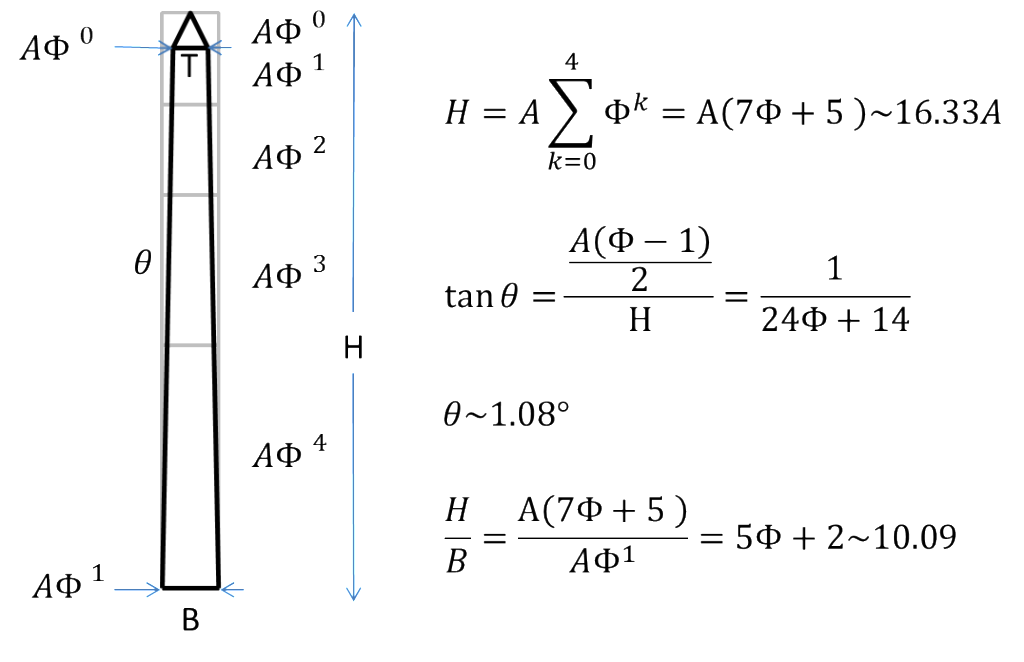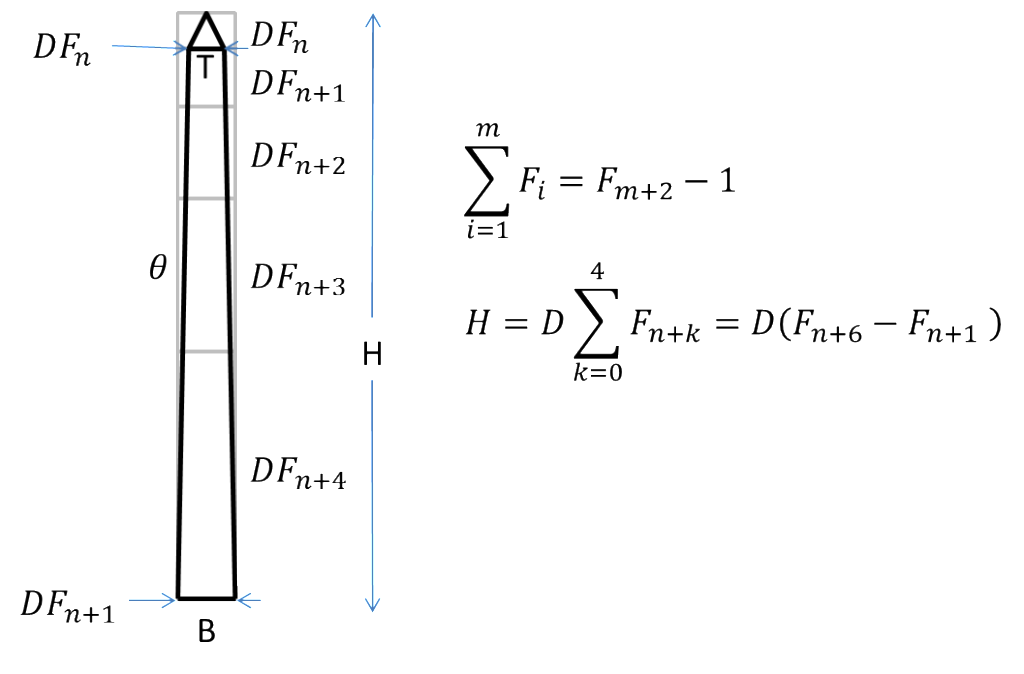# 02.02.06: Obelisk

## Example

Sources: Wikipedia and Wikipedia

## Where to Use

This form provides a strong vertical element that invokes a classic Egyptian architecture, often thought to be related to the sun.

## AbstractionThis is an example of Crepuscular Rays (Saint Peter’s Basilica Source: Wikipedia), which have an apparent angle of ~1.25 degrees (due to perspective). Notice the similarity to an obelisk.This is an example of a Sun Pillar (Source: Wikipedia) formed by the reflection of sun rays from plate-shaped ice crystals, usually seen when the sun is low to the horizon. The sun pillar can also extend downward. It has been proposed that these are the inspiration for obelisks. Note that the apparent angle of the pillar is approximately 1-2 degrees.

While exact dimensional proportions of surviving ancient obelisks vary, a good set of rules for creating the classical obelisk form can be established.

An interesting reference is John Bell’s paper “Some remarks on the application of Definite Proportion and the Conic Sections to Architecture, illustrated chiefly by the Obelisk, with some of that feature of Art” (Journal of the Society of Art Vol. 7 presented 25-May-1859). He presents a dimensional guide:

1. The diagonal of the base of the pyramidion (small pyramid on the top of the obelisk) is equal to the base of the obelisk.
2. The height of the pyramidion is equal to the base of the obelisk.
3. The overall height (including the pyramidion) is  7 times the diagonal of the obelisk base.

The last guide leads to the overall height (H) being approximately 10 times the base of the obelisk (B): H ~ 10B

The Washington Monument obelisk follows guidelines #2 and #3, but instead of the guideline #1 pyramidion base (T) to obelisk base (B) ratio of T ~ 0.707B, uses an approximate ratio of T ~ 0.625B. This increases the slope of the obelisk vertical section from ~0.94  to ~1.2 degrees.

Noting that the ratio of B/T is approximately the Golden Ratio, here is a set of guidelines for a Phi-based obelisk (Phi ~1.618), i.e. a “Golden Obelisk”:

1. The base of the obelisk (B) is Phi times the base of the pyramidion (T): B = Phi * T
2. The height of the pyramidion is equal to the base of the pyramidion (T).
3. The total height of the obelisk the sum of the first 5 terms in the Fibonacci sequence generated with the pyramidion base T and obelisk base B (see figure below)

This gives a shallower point on the end of the obelisk but the overall height (H) is H ~ 10.09B, similar to the Bell obelisk. The slope of the vertical section is ~1.08 degrees. The following drawing shows a Golden Obelisk.This form for the obelisk is approximated closely by using a construction based on a Fibonacci sequence as shown below (especially when n > 5):The appeal of this construction is the way each piece is related by a number in a Fibonacci sequence- it is a practical method for constructing the obelisk form to ‘classical’ proportions. Note that it also gives a natural way (with attractive scaling) to break up the face of the obelisk shaft into sections that might have different finishes or inscriptions.